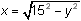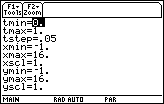Module 14 - Related Rates Introduction | Lesson 1 | Lesson 2 | Lesson 3 | Lesson 4 | Self-Test Lesson 14.2: The Ladder Problem With Gravity The ladder problem investigated in Lesson 14.1 is extended in this lesson to include the force of gravity on the ladder as it slides down the wall. Suppose the fifteen-foot ladder from Lesson 14.1 is held against a wall and the top of the ladder is being pulled downward by the force of gravity. The equation below models the position of the top of the ladder where the term -16t2 is the motion of the top of the ladder due to gravity and 15 represents the position of the top at time t = 0 seconds. y = –16t2 + 15 The equation for the position of the bottom of the ladder can be found by using the Pythagorean theorem.14.2.1 What are the parametric equations that model the position of the top and bottom of the ladder at time t? Click here for the answer. Displaying the Model Enter the parametric equations found in Question 14.2.1 into the Y= Editor Choose "Animate" for the Graph Style for both pairs of equations Make sure the Graph Order is still "Simultaneous" Use the Viewing Window values shown belowGraph the equations 14.2.2 Describe the apparent motion of the top and bottom of the ladder. Click here for the answer. 14.2.3 How fast is the bottom of the ladder moving at t = 0.2, t = 0.5 and t = 0.8 seconds? Click here for the answer. < Back | Next > ©Copyright 2007 All rights reserved. | Trademarks | Privacy Policy | Link Policy